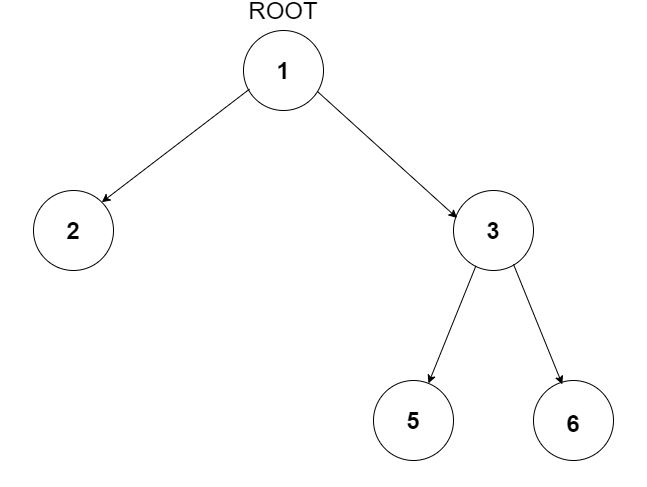# Minimum Depth Of Binary Tree

Posted: 4 Sep, 2020
Difficulty: Moderate

## PROBLEM STATEMENT

#### You have been given a Binary Tree of integers, find the minimum depth of this Binary Tree. The minimum depth of a Binary Tree is the number of nodes along the shortest path from the root node down to the nearest leaf node.

##### Note:
``````A leaf is a node with no children.
``````
##### For example:
``````For the given binary tree
````````````Output: 2
The shortest path is from root node 1 to leaf node 2 which contains 2 nodes in the path. Hence, the minimum depth is 2.
``````
##### Input Format:
``````The first line contains an integer 'T' which denotes the number of test cases or queries to be run. Then the test cases follow.

The only line of each test case contains elements in the level order form. The line consists of values of nodes separated by a single space. In case a node is null, we take -1 in its place.

For example, the input for the tree depicted in the below image would be :
````````````1
2 3
4 -1 5 6
-1 7 -1 -1 -1 -1
-1 -1
``````

#### Explanation :

``````Level 1 :
The root node of the tree is 1

Level 2 :
Left child of 1 = 2
Right child of 1 = 3

Level 3 :
Left child of 2 = 4
Right child of 2 = null (-1)
Left child of 3 = 5
Right child of 3 = 6

Level 4 :
Left child of 4 = null (-1)
Right child of 4 = 7
Left child of 5 = null (-1)
Right child of 5 = null (-1)
Left child of 6 = null (-1)
Right child of 6 = null (-1)

Level 5 :
Left child of 7 = null (-1)
Right child of 7 = null (-1)

The first not-null node (of the previous level) is treated as the parent of the first two nodes of the current level. The second not-null node (of the previous level) is treated as the parent node for the next two nodes of the current level and so on.
The input ends when all nodes at the last level are null (-1).
``````
##### Note :
``````The above format was just to provide clarity on how the input is formed for a given tree.

The sequence will be put together in a single line separated by a single space. Hence, for the above-depicted tree, the input will be given as:

1 2 3 4 -1 5 6 -1 7 -1 -1 -1 -1 -1 -1
``````
##### Output Format:
``````For each test case:

Print an integer i.e. Minimum depth of the BInary Tree.

Print output of each test case in a separate line.
``````
##### Note:
``````You are not required to print the expected output, it has already been taken care of. Just implement the function.
``````
##### Constraints:
``````1 <= T <= 10^2
0 <= N <= 10^3

Time Limit: 1 sec
``````Approach 1

We can write a recursive solution for finding the minimum depth in a Binary Tree. The idea here is to first find the minimum depths of left and right subtrees and then the minimum depth of the current node will be 1 + min(minDepthLeftSubtree, minDepthRightSubtree).

Here is the complete algorithm:

• Traverse in given Binary Tree.
• If currentNode is NULL, terminate recursion (return 0)
• If currentNode is a leaf node, return 1.
• If currentNode only has a left child, return 1 + the minimum depth of the left subtree.
• If currentNode only has a right child, return 1 + the minimum depth of the right subtree.
• If currentNode has both children, find the minimum depth of both subtrees and return 1 + min(minDepthLeftSubtree, minDepthRightSubtree).